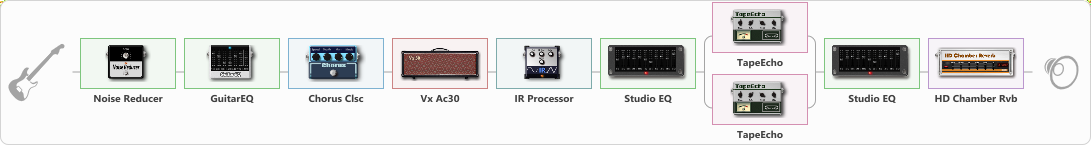# Spot Groove Truss

Discussion in 'ToneLib-GFX presets' started by truss van halen, May 8, 2021.

1. ### truss van halenWell-Known Member

Spot Groove Truss

Preset name: Spot groove Truss

Effects chain:Effect: "Noise Reducer" (Dynamics / Filter), active - "yes"
{
"Sens" = 98
"Mode" = Hard
}

Effect: "GuitarEQ" (Dynamics / Filter), active - "yes"
{
"160 Hz" = 0
"400 Hz" = 8
"800 Hz" = 0
"1.6 kHz" = 0
"3.2 kHz" = 5
"6.4 kHz" = 0
"12 kHz" = 0
"Level (dB)" = 0
}

Effect: "Chorus Clsc" (Modulation / Sfx), active - "yes"
{
"Speed" = 3.5
"Depth" = 58
"Center" = 1.0
"Mode" = Stereo
}

Effect: "Vx Ac30" (Amp simulators), active - "yes"
{
"Gain" = 8
"Bass" = 30
"Middle" = 90
"Treble" = 39
"Presence" = 50
"Master" = 80
"Level (dB)" = 10
}

Effect: "IR Processor" (Cabinets), active - "yes"
{
"IR" = Vox AC30
"Low Cut (Hz)" = 57
"Hi Cut (kHz)" = 7.5
"Mix" = 100
"Level (dB)" = 0
}

Effect: "Studio EQ" (Dynamics / Filter), active - "yes"
{
"31 Hz" = -15
"62 Hz" = -15
"125 Hz" = -7
"250 Hz" = 2
"500 Hz" = 3
"1 kHz" = -3
"2 kHz" = 5
"4 kHz" = 6
"8 kHz" = 6
"16 kHz" = -4
"Level (dB)" = 0
}

Effect: "Splitter" (Dynamics / Filter)
{
"A-Bypass" = Off
"A-Pan" = 0
"A-Level" = 55
"B-Bypass" = Off
"B-Pan" = 0
"B-Level" = 55

'A' branch:
{

Effect: "TapeEcho" (Delay), active - "yes"
{
"Time" = 100
"Feedback" = 0
"Tone" = 47
"LoDamp" = 35
"Mix" = 57
}
}
'B' branch:
{

Effect: "TapeEcho" (Delay), active - "yes"
{
"Time" = 200
"Feedback" = 58
"Tone" = 65
"LoDamp" = 19
"Mix" = 72
}
}
}

Effect: "Studio EQ" (Dynamics / Filter), active - "yes"
{
"31 Hz" = -14
"62 Hz" = -11
"125 Hz" = 4
"250 Hz" = 5
"500 Hz" = -6
"1 kHz" = -1
"2 kHz" = 8
"4 kHz" = 6
"8 kHz" = -12
"16 kHz" = -12
"Level (dB)" = 0
}

Effect: "HD Chamber Rvb" (Reverberation), active - "yes"
{
"Time" = 0.8
"PreLPF" = 10
"PreDelay" = 71
"HiDamp" = 0
"LoGain" = 1.5
"Mix" = 6
}

Note: You will need to download and install the ToneLib-GFX software to use the preset.

#### Attached Files:

• ###### Spot_Groove_Truss.tlgfx
File size:
1.5 KB
Views:
2,563
Onur, SEMENOV and ktaxi like this.